##### The Japanese Bonsai specialist
Direct order Contact Help / Services Newsletter# Hosta 'blue mouse ears'

Giboshi blue mouse ears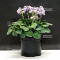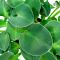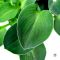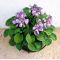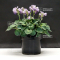ref. : 10501

14,00

Available quantity : 9Order

###### Description

Large rhizome delivered in a 3 litre plastic pot. Hosta 'Blue Mouse Ears' produces small round dark aquamarine leaves that become a beautiful green dark in summer. Mauve pink flowers abound at the beginning of July. This plant is also a good kusamono accompaniment for bonsai.

Height: 20 cm x 50 cm wide.

Leaves 8/10 cm long.

Derived from a 'Blue Cadet' hosta seedling, 2000.

Awarded Hosta of the Year 2008 by the American Hosta Growers Association.

Good resistance against slugs and snails.

#hosta 6.5 #blue 4.4 #mouse 3.5 #ears 3.4 #hostas 2.6 #leaves 2.6 #good 2.4 #dark 2.4 #accompaniment 2.3 #association 2.1

Formule
(( ROUND((CHAR_LENGTH(b.article_nom)-CHAR_LENGTH(REPLACE(b.article_nom, 'hosta', '')))/LENGTH('hosta')) + ROUND((CHAR_LENGTH(b.article_description)-CHAR_LENGTH(REPLACE(b.article_description, 'hosta', '')))/LENGTH('hosta')) ) * 6.5) + (( ROUND((CHAR_LENGTH(b.article_nom)-CHAR_LENGTH(REPLACE(b.article_nom, 'blue', '')))/LENGTH('blue')) + ROUND((CHAR_LENGTH(b.article_description)-CHAR_LENGTH(REPLACE(b.article_description, 'blue', '')))/LENGTH('blue')) ) * 4.4) + (( ROUND((CHAR_LENGTH(b.article_nom)-CHAR_LENGTH(REPLACE(b.article_nom, 'mouse', '')))/LENGTH('mouse')) + ROUND((CHAR_LENGTH(b.article_description)-CHAR_LENGTH(REPLACE(b.article_description, 'mouse', '')))/LENGTH('mouse')) ) * 3.5) + (( ROUND((CHAR_LENGTH(b.article_nom)-CHAR_LENGTH(REPLACE(b.article_nom, 'ears', '')))/LENGTH('ears')) + ROUND((CHAR_LENGTH(b.article_description)-CHAR_LENGTH(REPLACE(b.article_description, 'ears', '')))/LENGTH('ears')) ) * 3.4) + (( ROUND((CHAR_LENGTH(b.article_nom)-CHAR_LENGTH(REPLACE(b.article_nom, 'leaves', '')))/LENGTH('leaves')) + ROUND((CHAR_LENGTH(b.article_description)-CHAR_LENGTH(REPLACE(b.article_description, 'leaves', '')))/LENGTH('leaves')) ) * 2.6) + (( ROUND((CHAR_LENGTH(b.article_nom)-CHAR_LENGTH(REPLACE(b.article_nom, 'good', '')))/LENGTH('good')) + ROUND((CHAR_LENGTH(b.article_description)-CHAR_LENGTH(REPLACE(b.article_description, 'good', '')))/LENGTH('good')) ) * 2.4) + (( ROUND((CHAR_LENGTH(b.article_nom)-CHAR_LENGTH(REPLACE(b.article_nom, 'dark', '')))/LENGTH('dark')) + ROUND((CHAR_LENGTH(b.article_description)-CHAR_LENGTH(REPLACE(b.article_description, 'dark', '')))/LENGTH('dark')) ) * 2.4) + (( ROUND((CHAR_LENGTH(b.article_nom)-CHAR_LENGTH(REPLACE(b.article_nom, 'accompaniment', '')))/LENGTH('accompaniment')) + ROUND((CHAR_LENGTH(b.article_description)-CHAR_LENGTH(REPLACE(b.article_description, 'accompaniment', '')))/LENGTH('accompaniment')) ) * 2.3) + (( ROUND((CHAR_LENGTH(b.article_nom)-CHAR_LENGTH(REPLACE(b.article_nom, 'association', '')))/LENGTH('association')) + ROUND((CHAR_LENGTH(b.article_description)-CHAR_LENGTH(REPLACE(b.article_description, 'association', '')))/LENGTH('association')) ) * 2.1) + (( ROUND((CHAR_LENGTH(b.article_nom)-CHAR_LENGTH(REPLACE(b.article_nom, 'aquamarine', '')))/LENGTH('aquamarine')) + ROUND((CHAR_LENGTH(b.article_description)-CHAR_LENGTH(REPLACE(b.article_description, 'aquamarine', '')))/LENGTH('aquamarine')) ) * 2)

## Secure payment## Delivery

Our logistic partners :